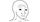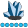somebody
Lapis Lazuli | Level 10

## Return computation with PROC EXPAND

I would like to calculate returns using PROC EXPAND. I could use just a simple DATA step but I would like to learn more about PROC EXPAND.

My dataset has 3 columns containing stock, date, and price. I could use the following DATA step:

``````data stocks;
set stocks;
by stock date ;
return=price/lag(price)-1;
if first.stock then return=.;
run;``````

But How do I do  this using PROC EXPAND? in particular, what do I put in the TRANSFORMIN and TRANSFORMOUT ?

Thanks

1 ACCEPTED SOLUTION

Accepted Solutions

## Re: Return computation with PROC EXPAND

``````proc sort data=stocks;
by stock date;
run;
proc expand data=stocks out=result(drop=time);
by stock;
convert price=return / transformout=( ratio 1 -1);
run;``````
3 REPLIES 3

## Re: Return computation with PROC EXPAND

``````proc sort data=stocks;
by stock date;
run;
proc expand data=stocks out=result(drop=time);
by stock;
convert price=return / transformout=( ratio 1 -1);
run;``````somebody
Lapis Lazuli | Level 10

## Re: Return computation with PROC EXPAND

Thanks. just to confirm if I understand the code correctly,

``transformout=( ratio 1 -1)``

means getting the ratio of the current value over lag 1 and then minus 1 to get the return right? So, say if I want to get returns between now and 4 periods ago, it should be:

``transformout=( ratio 4 -1)``

Am I correct?

## Re: Return computation with PROC EXPAND

well done

Discussion stats
• 3 replies
• 680 views
• 2 likes
• 2 in conversation